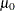### OUT= Output Data Set in the OUTPUT Statement

PROC UNIVARIATE creates an OUT= data set for each OUTPUT statement. This data set contains an observation for each combination of levels of the variables in the BY statement, or a single observation if you do not specify a BY statement. Thus the number of observations in the new data set corresponds to the number of groups for which statistics are calculated. Without a BY statement, the procedure computes statistics and percentiles by using all the observations in the input data set. With a BY statement, the procedure computes statistics and percentiles by using the observations within each BY group.

The variables in the OUT= data set are as follows:

• BY statement variables. The values of these variables match the values in the corresponding BY group in the DATA= data set and indicate which BY group each observation summarizes.

• variables created by selecting statistics in the OUTPUT statement. The statistics are computed using all the nonmissing data, or they are computed for each BY group if you use a BY statement.

• variables created by requesting new percentiles with the PCTLPTS= option. The names of these new variables depend on the values of the PCTLPRE= and PCTLNAME= options.

If the output data set contains a percentile variable or a quartile variable, the percentile definition assigned with the PCTLDEF= option in the PROC UNIVARIATE statement is recorded in the output data set label. See Example 4.8.

The following table lists variables available in the OUT= data set.

Table 4.36: Variables Available in the OUT= Data Set

Variable Name

Description

Descriptive Statistics

CSS

sum of squares corrected for the mean

CV

percent coefficient of variation

KURTOSIS | KURT

measurement of the heaviness of tails

MAX

largest (maximum) value

MEAN

arithmetic mean

MIN

smallest (minimum) value

MODE

most frequent value (if not unique, the smallest mode)

N

number of observations on which calculations are based

NMISS

number of missing observations

NOBS

total number of observations

RANGE

difference between the maximum and minimum values

SKEWNESS | SKEW

measurement of the tendency of the deviations to be larger in one direction than in the other

STD | STDDEV

standard deviation

STDMEAN | STDERR

standard error of the mean

SUM

sum

SUMWGT

sum of the weights

USS

uncorrected sum of squares

VAR

variance

Quantile Statistics

MEDIAN | Q2 | P50

middle value (50th percentile)

P1

1st percentile

P5

5th percentile

P10

10th percentile

P90

90th percentile

P95

95th percentile

P99

99th percentile

Q1 | P25

lower quartile (25th percentile)

Q3 | P75

upper quartile (75th percentile)

QRANGE

difference between the upper and lower quartiles (also known as the inner quartile range)

Robust Statistics

GINI

Gini’s mean difference

median absolute difference

QN

2nd variation of median absolute difference

SN

1st variation of median absolute difference

STD_GINI

standard deviation for Gini’s mean difference

standard deviation for median absolute difference

STD_QN

standard deviation for the second variation of the median absolute difference

STD_QRANGE

estimate of the standard deviation, based on interquartile range

STD_SN

standard deviation for the first variation of the median absolute difference

Hypothesis Test Statistics

MSIGN

sign statistic

NORMAL

test statistic for normality. If the sample size is less than or equal to 2000, this is the Shapiro-Wilk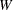statistic. Otherwise, it is the Kolmogorov
statistic.

PROBM

probability of a greater absolute value for the sign statistic

PROBN

probability that the data came from a normal distribution

PROBS

probability of a greater absolute value for the signed rank statistic

PROBT

two-tailed
-value for Student’sstatistic with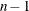degrees of freedom

SIGNRANK

signed rank statistic

T

Student’sstatistic to test the null hypothesis that the population mean is equal to